# DAC - Static Parameters

Digital to Analog Converter (ADC) is a device to converts a sequence of digital input data into analog signals.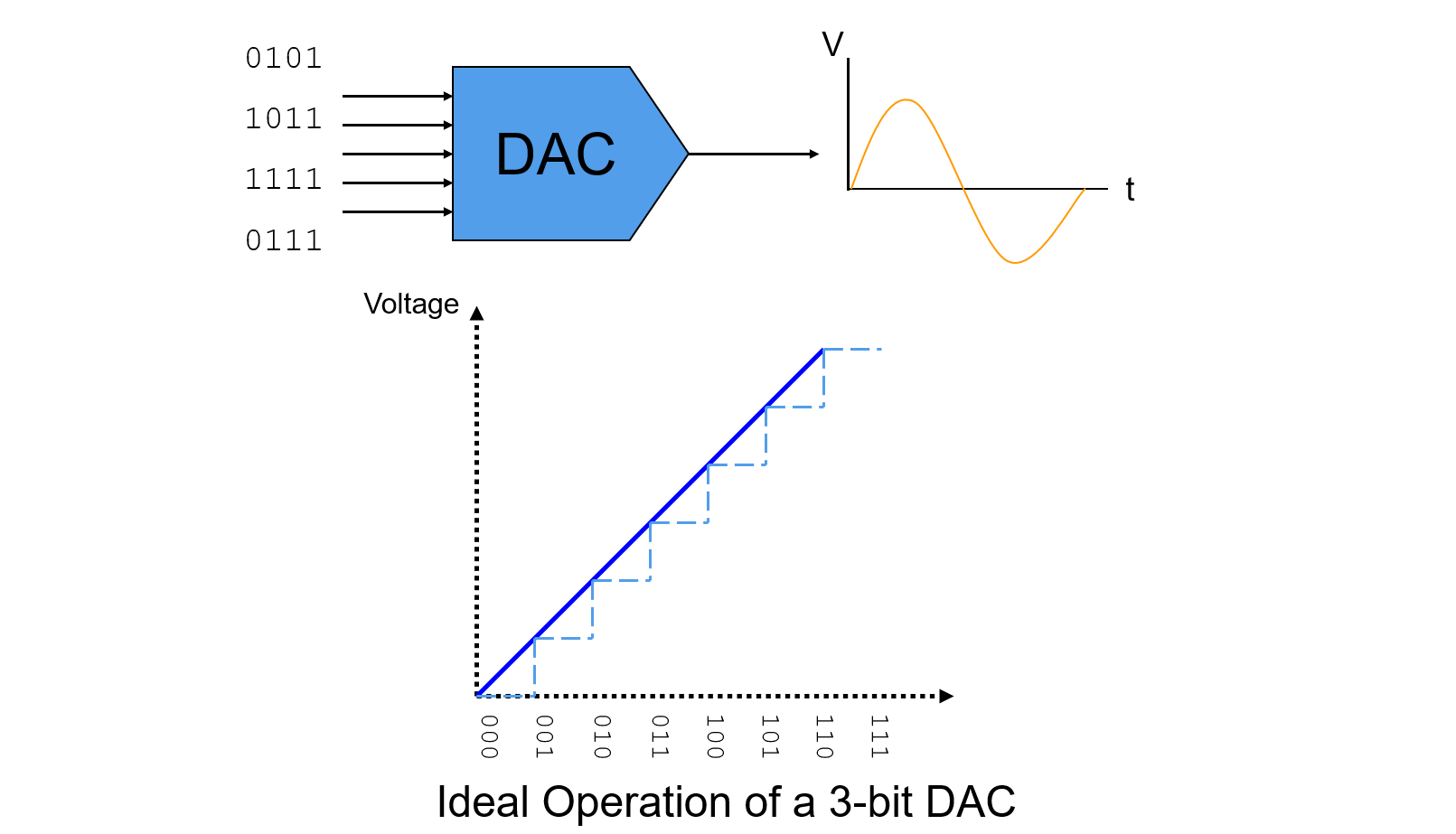## Static Parameters

DAC's static parameters mainly contain:

• Zero Scale Output
• Full Scale Range (FSR)
• LSB Size
• Offset Error
• Gain Error
• Differential Non-Linearity Error (DNE or DNL)
• Integral Non-Linearity Error (INE or INL)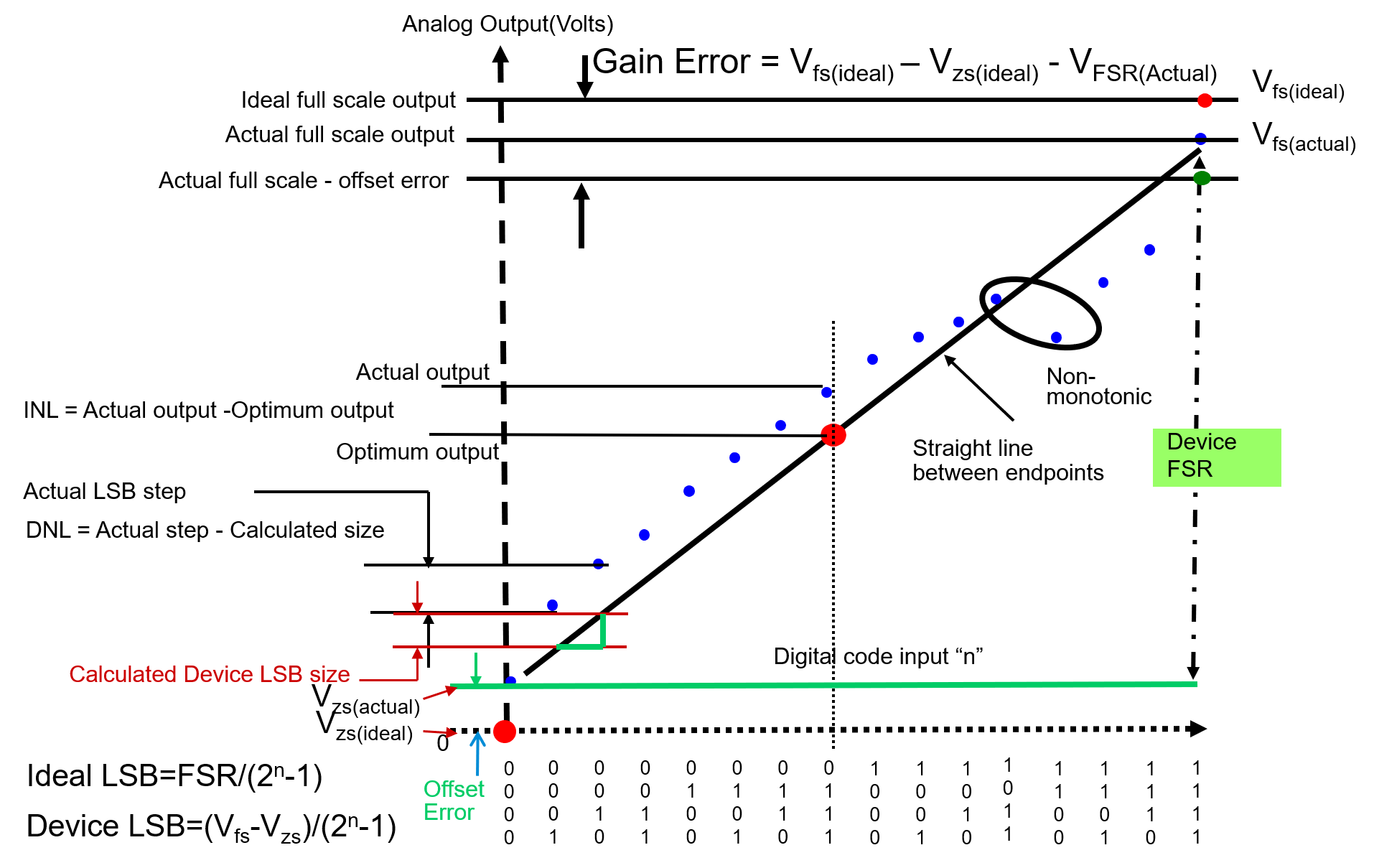### Zero Scale Output

Zero Scale Output is the measured output value when the zero/null level digital input code is presented to the DUT.

### Full Scale Range (FSR)

Range of DAC output voltage between the minimum ($$V_{ZS}$$) and maximum ($$V_{FS}$$) analog outputs is called Full Scale Range (FSR):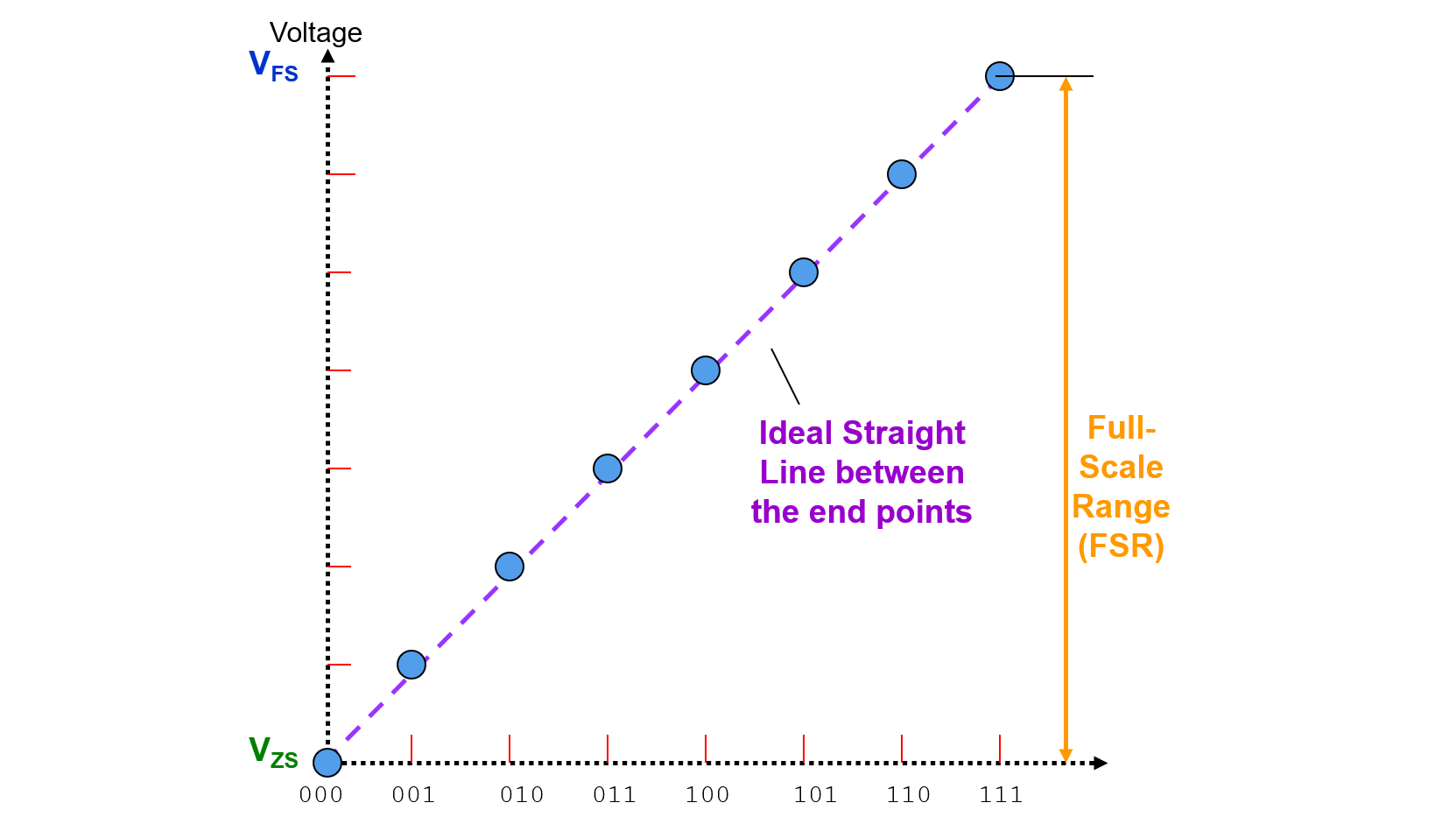### LSB Size

Average change in voltage when in between the input codes is defined as LSB:

$LSB=\frac{FSR_{measured}}{2^{bits}-1}$

### Offset Error

Offset Error (Zero-Scale Error) is the voltage difference between ideal and actual offset (initial) points.

$OffsetError=V_{ZS(Actual)}-V_{ZS(ideal)}$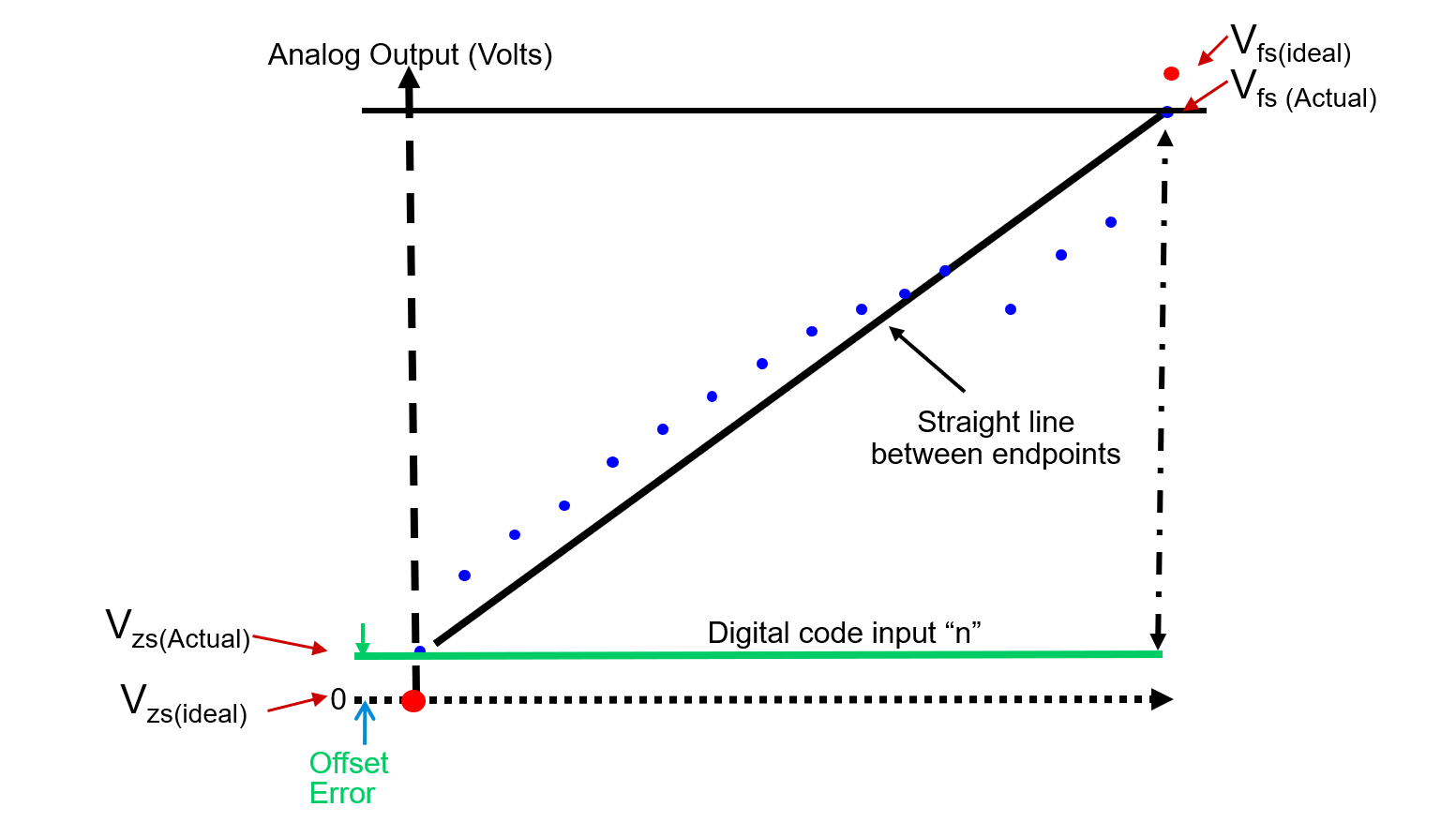### Gain Error

Gain Error is the voltage difference between ideal and actual gain points on the transfer function.

$GainError=FSR_{Ideal}-FSR_{Actual}$

Where

$FSR_{Ideal}=V_{FS(ideal)}-V_{ZS(ideal)}$
$FSR_{Actual}=V_{FS(Actual)}-V_{ZS(Actual)}$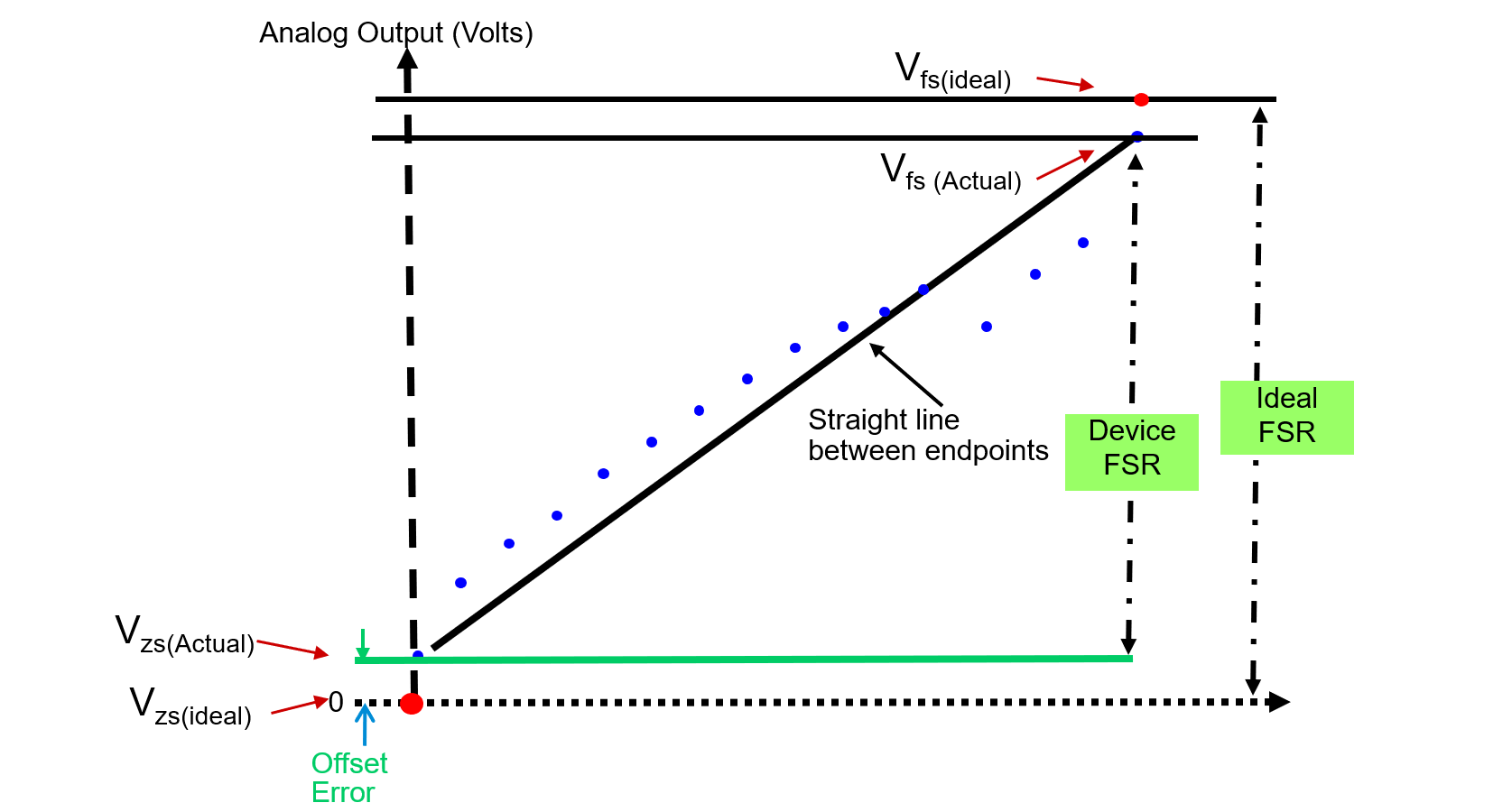### Differential Non-Linearity Error (DNL)

Differential Non-Linearity Error (DNL) is the difference in the output voltage at a specific, compared to the output at the previous input, then minus one device LSB:

$DNL=(V_{in2}-V_{in1})-LSB_{average}$

where $$V_{in2}$$ is the voltage of the upper transition, $$V_{in1}$$ is the lower.

DNL is a measure of "small-signal" linearity error. Measurement of DNL is made from one step to the next, not each step to the ideal value.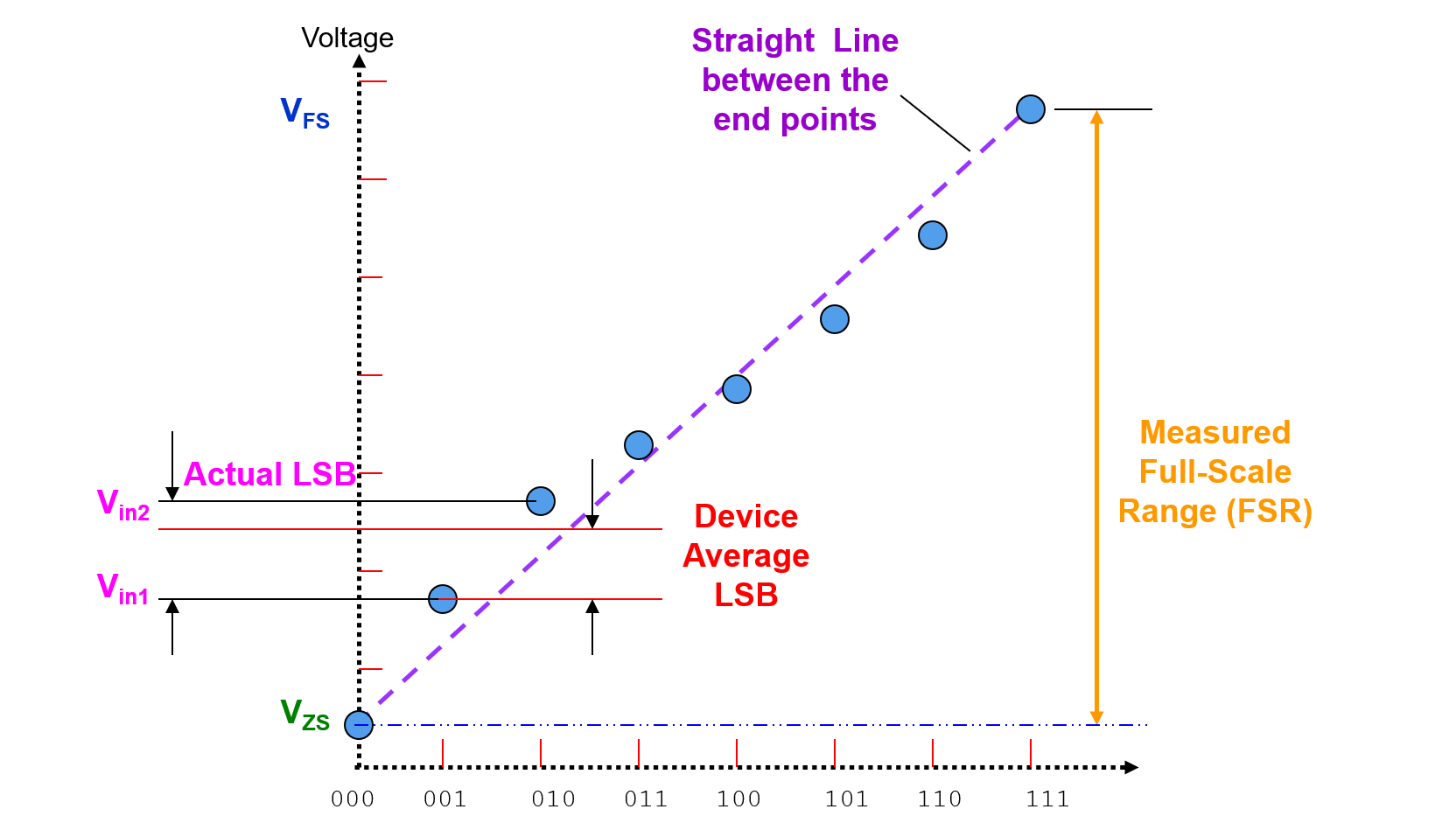### Integral Non-Linearity Error (INL)

Integral Non-Linearity Error (INL) is the cumulative effect of all differential non-linearity values.It is a measure of "large-signal" linearity error. INL at any point along the curve is the deviation of the ideal linearity line.

$ExpectedOutput[i]=FSR*InputCode[i]+OffsetError$
$INL[i]=\frac{ActualOutput[i]-ExpectedOutput[i]}{LSB_{average}}$

Also, INL can also be expressed as a function of DNL:

$INL[i]=\sum_{n=1}^{n=i}DNL[n]$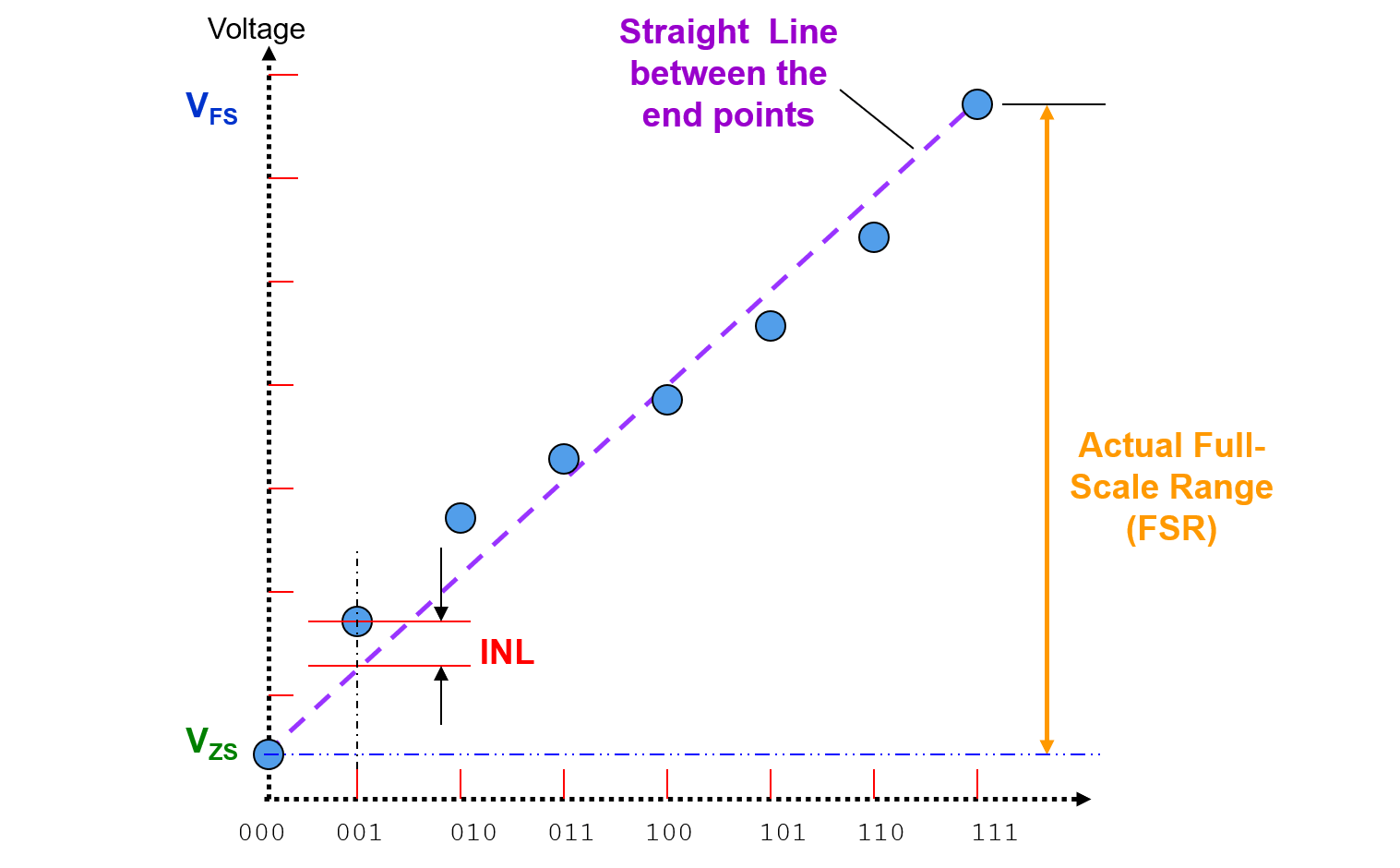## How to Test Static Parameters

### Test System Setup

Test system setup for DAC static parameter tests: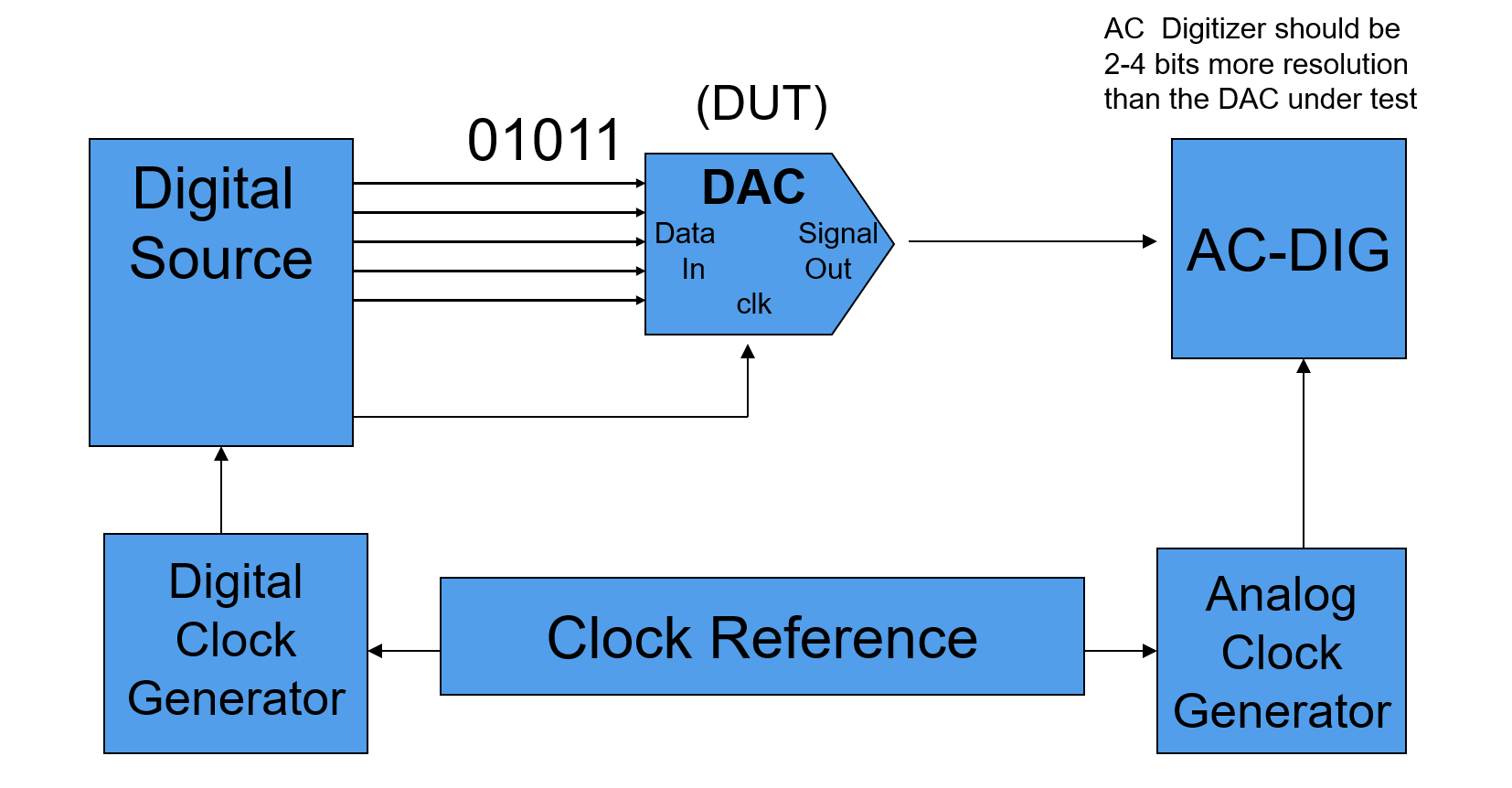Block diagram of signal setup: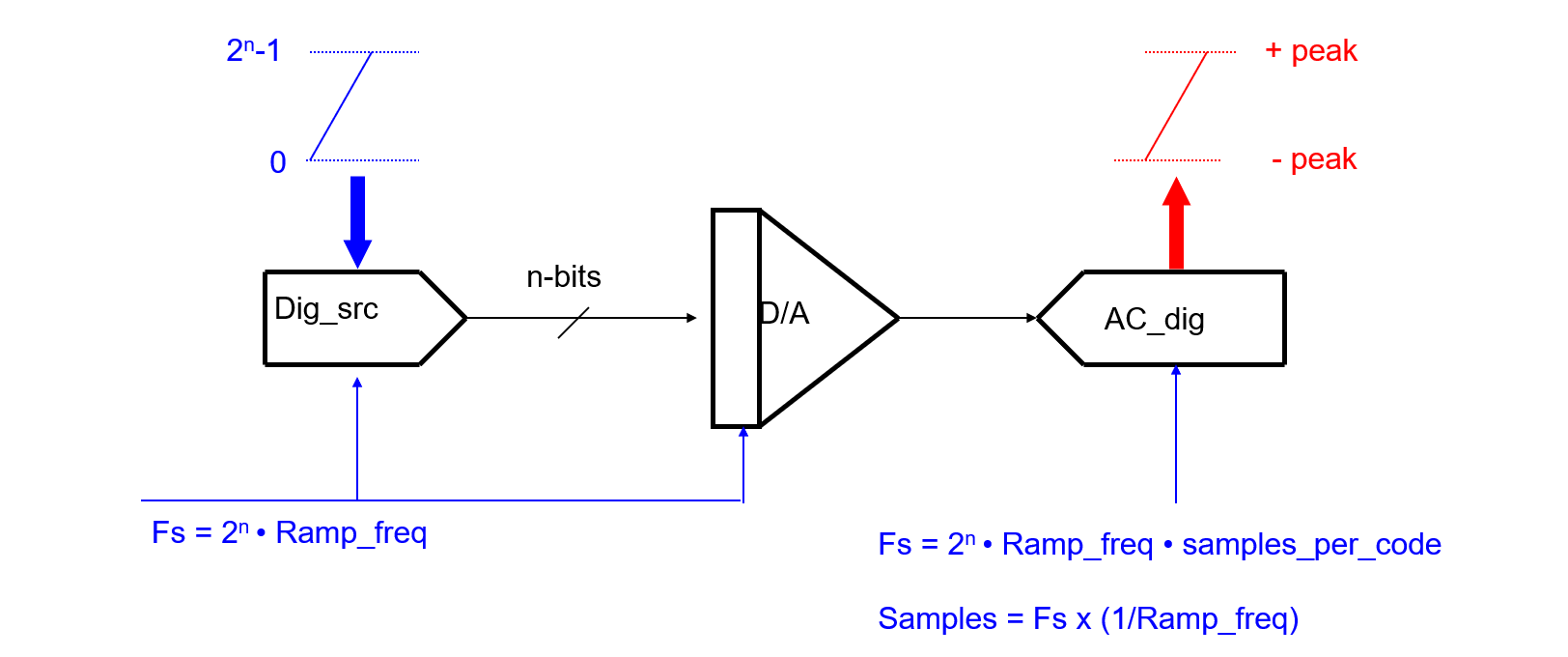### Tests Concept

Procedure of testing the static parameters of an DAC DUT is listed below.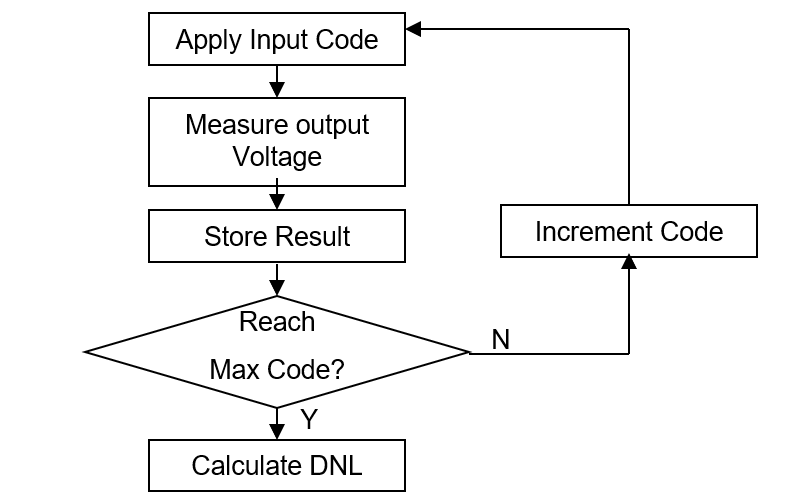#### 1. Measure the output voltage by applying the digital data inputs from Zero Scale to Full Scale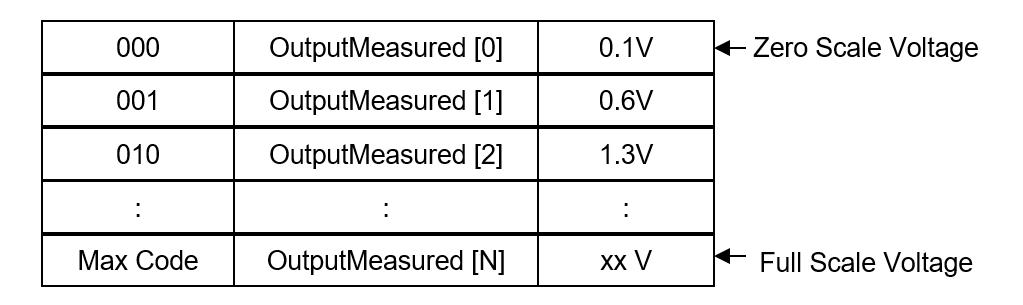#### 2. Calculate DNL for each input code

$DNL[i]=\frac{OutputMeasured[i]-OutputMeasured[i-1]-LSB_{average}}{LSB_{average}}$

Where

$LSB_{average}=\frac{OutputMeasured[n]-OutputMeasured}{2^{bits}-1}$

## References & Acknowledgements

• Fundamentals of Testing Using ATE
• The-Fundamentals-of-Mixed-Signal-Testing_Brian-Lowe

Original: https://wiki-power.com/
This post is protected by CC BY-NC-SA 4.0 agreement, should be reproduced with attribution.